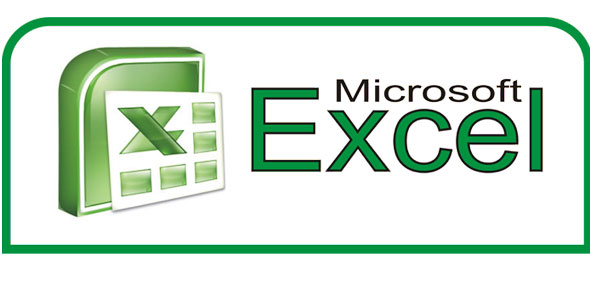# Excel Quiz 1

7 Questions | Total Attempts: 1637SettingsThis test can be used as a pre-test assessment of student knowledge or as a post-lesson test for student comprehension. If you need a refresher before beginning this quiz, go to: http://docs. Google. Com/EmbedSlideshow? Docid=ds6m4nn_3f8cnr74c

Related Topics
• 1.
In order to save a new document in Microsoft Excel you must select which one of the following tool bar options?
• A.

Edit

• B.

Format

• C.

Help

• D.

File

• 2.
An Excel spreadsheet is primarily used for calculating which of the following options?
• A.

Data

• B.

Finances

• C.

Numbers

• D.

All of the above

• 3.
Which of the following option is a formula?
• A.

=SUM(A1:A5)

• B.

• C.

Subtract the numbers from A1 to A5

• D.

A1 = A5

• 4.
Which one of the following options CANNOT be used in an Excel spreadsheet formula?
• A.

= (equal sign)

• B.

, (comma)

• C.

& (ampersand)

• D.

: (colon)

• 5.
What is the function of the word '=SUM' at the beginning of an Excel spreadsheet formula?
• A.

• B.

To tell the person viewing that this is a function and it should be added together

• C.

To calculate all the data correctly without any mistakes

• D.

To inform the computer that an arithmetic function will occur

• 6.
Which of the following INCORRECTLY selects multiple cells?
• A.

(A1:G50)

• B.

(A1, B3:C9)

• C.

(A1:B5:C5)

• D.

(A1:B5#C5)

• 7.
In order to add or alter a formula, under which of the following menu options can the 'function' menu be found?
• A.

Tools

• B.

File

• C.

Help

• D.

Insert